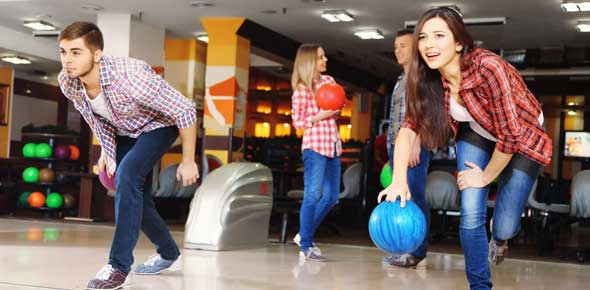# The Candid Test On Bowling Lesson 6

29 Questions | Total Attempts: 334SettingsThere are some people who are so good at bowling such that they rarely loose to other people and if you want to play n the big league the lessons we undertake will get you there. Take up this candid test on bowling from lesson six and see how much you know.

Related Topics
• 1.
Where should you aim when attempting to pick up the #10 pin?
• A.

A. directly opposite the pin

• B.

B. to the left of center

• C.

C. in the center of the approach

• D.

D. facing the third arrow on the right

• 2.
The following spare is called a "sleeper":
• A.

A. 2-8

• B.

B. 4-8

• C.

C. 3-4

• D.

D. 1-2

• 3.
The name "sleeper" means
• A.

A. three pins are left

• B.

B. one pin is hidden directly behind another pin

• C.

C. one half of the pins are left

• D.

D. two pins are left, one in the middle and one to the side

• 4.
A ball which curves or "fades away" to the right for a right-handed bowler is called
• A.

A. a curve ball

• B.

B. a hook ball

• C.

C. a backup ball or reverse hook

• D.

D. a straight ball

• 5.
In spot bowling, the target arrow to watch
• A.

A. never changes

• B.

B. is at the far end of the alley

• C.

C. is an arrow approximately 16 feet from the foul line

• D.

D. is on the ball

• 6.
The reason for advocating that you use the spot method of aiming is
• A.

A. All of these

• B.

B. that it is highly recommended by the experts

• C.

C. that the target is so much closer than the pins

• D.

D. reliable statistics

• 7.
All of the pin numbers contacted by the ball on a perfect 1-3 pocket hit would be
• A.

A. 1-3-5-8-9

• B.

B. 1-3-5-8

• C.

C. 1-3-5-9

• D.

D. 1-2-6-10

• 8.
A situation occurs where pins were left standing after two balls have been delivered. What is this called?
• A.

A. a split

• B.

B. a miss

• C.

C. a cherry

• D.

D. a sweeper

• 9.
After the first ball, two pins remain standing with no intermediate pins in front or between them. (The head pin is down.) What is this called?
• A.

A. a cherry

• B.

B. a sweeper

• C.

C. an error

• D.

D. a split

• 10.
The pins remaining after the first ball is rolled in a frame are called
• A.

A. cherry

• B.

• C.

C. leave

• D.

D. bucket

• 11.
The symbol for a split is
• A.

A. "“

• B.

B. x

• C.

C. O

• D.

D. /

• 12.
The symbol for a miss is
• A.

A. "“

• B.

B. /

• C.

C. O

• D.

D. x

• 13.
Which of the following is true if a "foul" occurs?
• A.

A. Nothing happens

• B.

B. The ball does not return

• C.

C. The pins fall down.

• D.

D. A buzzer sounds or a light comes on or both

• 14.
Using actual scores without handicaps is called
• A.

A. scratch

• B.

B. split

• C.

C. steal

• D.

D. spare

• 15.
The space between the 1 and 3 pins for a right-handed bowler is called
• A.

A. cherry

• B.

B. pocket

• C.

C. frame

• 16.
All pins down on the first ball constitutes a
• A.

A. spare

• B.

B. strike

• C.

C. back up

• D.

D. cherry.

• 17.
Score the first three frames
• A.

A. 49

• B.

B. 45

• 18.
The object is to hit the 1-3 pocket
• A.

A. true

• B.

B. false

• 19.
As a general rule, roll spares at cross angles.
• A.

A. true

• B.

B. false

• 20.
Taps are usually the result of poor ball action
• A.

A. true

• B.

B. false

• 21.
On a perfect hit, the ball only contacts 4 pins
• A.

A. true

• B.

B. false

• 22.
Most expert bowlers use the pins as their point of aim.
• A.

A. true

• B.

B. false

• 23.
Target arrows are the all-important "spots" in the spot bowling technique
• A.

A. true

• B.

B. false

• 24.
To pick up the 7 pin, a bowler should move the starting position slightly right and the point of aim to the left
• A.

A. true

• B.

B. false

• 25.
Most expert bowlers spot bowl the first ball and pin bowl the second ball
• A.

A. true

• B.

B. false